# 呰MindTap . Cengage L e The National Footbal 呰 xCengage | Cengage Cengage Digital Lear Cengage xCengage x ← → С 슬 ht...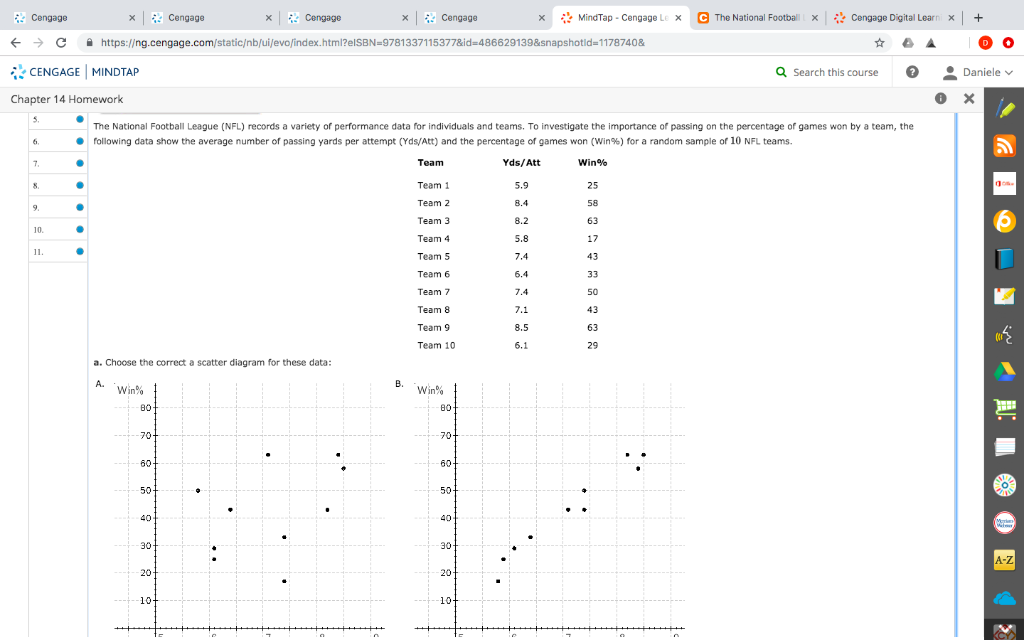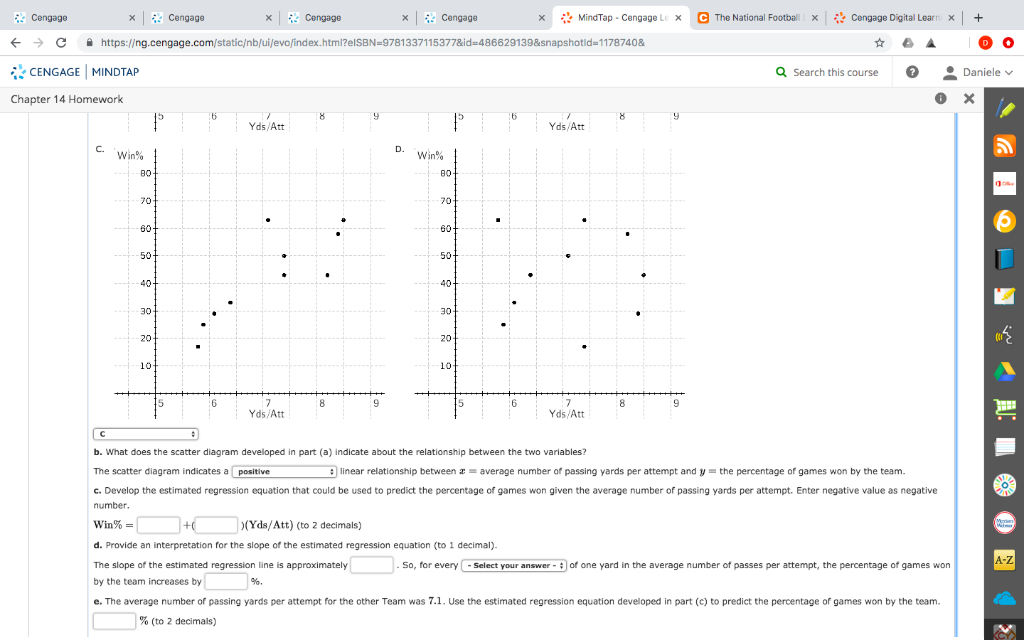(a) SCATTER DIAGRAM:

The correct scatter plot is Option (B):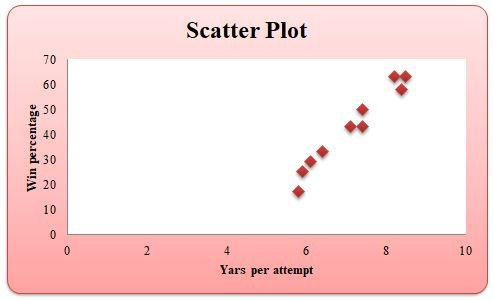(b) INTERPRETATION OF SCATTER PLOT:

The scatter diagram indicates a positive linear relationship between= average number of passing yards per attempt and= the percentage of games won by the team.

(c) SIMPLE LINEAR REGRESSION EQUATION:

I have used R code to build simple linear regression model to the given data.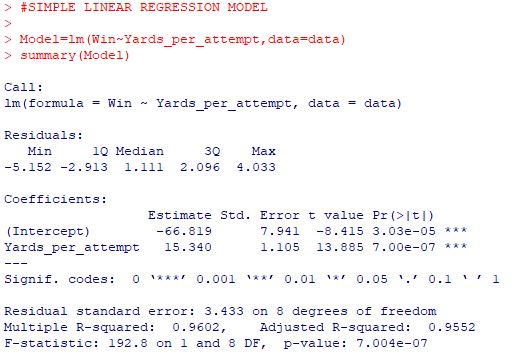The estimated simple linear regression equation is given by,is the predicted dependent variable "Win percentage".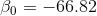is the intercept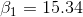is the slope coefficient of independent variable "Yards per attempt"is the independent variable "Yards per attempt".

(d) INTERPRETATION OF SLOPE OF ESTIMATED REGRESSION EQUATION:

The slope of the estimated regression equation is approximately. So, for every increase of one yard in the average number of passes per attempt, the percentage of games won by the team increases by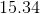%.

(e) PREDICTED PERCENTAGE OF GAMES WON BY THE TEAM:

The estimated simple linear regression equation is given by,If the average number of passing yard per attempt for the other team was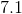, the predicted percentage of games won by the team is,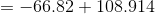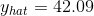If the average number of passing yard per attempt for the other team was, the predicted percentage of games won by the team is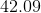%.

##### Add Answer of: 呰MindTap . Cengage L e The National Footbal 呰 xCengage | Cengage Cengage Digital Lear Cengage xCengage x ← → С 슬 ht...
More Homework Help Questions Additional questions in this topic.